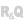HelpWLanguageWLanguage functionsControls, pages and windowsDiagramLoadLibraryPresentationExampleSee alsoDiagram constantsDiagram functionsDiagramEndModificationDiagramGroupDiagramInfoXYDiagramLoadDiagramLoadLibraryDiagramSaveDiagramSearchDiagramSelectMinusDiagramSelectPlusDiagramShapeToImageDiagramStartModificationDiagramToImageDiagramToSVGDiagramUngroup
 WINDEVWEBDEVWINDEV MobileOthersDiagramLoadLibrary (Function) In french: DiagrammeChargeBibliothèque Loads a diagram file and creates a library of preset shapes from it.Example // Add a new libraryMyLibrary is diagLibraryDiagramLoadLibrary(MyLibrary, "Furniture01.wddiag")MyLibrary.Name = "Furniture"DIAGEDT_Diagram.Library.Add(MyLibrary) sFile is string = fTempDir() + [ fSep ] + "custom_library.wddiag" // Creates a library entirely through programmingTempLibrary is Diagram D1 is diagOvalD1.Width = 50D1.Height = 50D1.Background.Color = DarkRedAdd(TempLibrary.Shape, D1) D2 is diagOvalD2.Width = 50D2.Height = 70D2.Background.Color = DarkGreenAdd(TempLibrary.Shape, D2) // Use the shapes of the temporary diagram to create the library// > saves the diagram to the diskDiagramSave(TempLibrary, sFile) // Load the diagram as a libraryMyLibrary is diagLibraryDiagramLoadLibrary(MyLibrary, sFile)MyLibrary.Name = "Custom" // Adds the library to the Diagram Editor controlAdd(DIAGEDT_Diagram.Library, MyLibrary) ToastDisplay("The custom library has been added to the list.") Syntax = DiagramLoadLibrary( , ) : BooleanTrue if the library has been loaded, False otherwise. ErrorInfo returns more details about the error. : diagLibrary variableName of the diagLibrary variable into which the file corresponding to the library must be imported. : Character stringFull path of the diagram file corresponding to the library to be imported. This file corresponds to a diagram previously saved with DiagramSave. Remarks A library corresponds to a diagram file created with DiagramSave (".wddiag" file). DiagramLoadLibrary transforms a diagram into a library of preset shapes that can be displayed in the left panel of the Diagram Editor control.To use only your custom libraries, delete the default libraries. To do so, use ArrayDeleteAll on the array of libraries of the diagram. Example: ArrayDeleteAll(DIAGEDT_Diagram.Library) Business / UI classification: UI CodeComponent: wd280mdl.dll Minimum version required Version 27 This page is also available for…